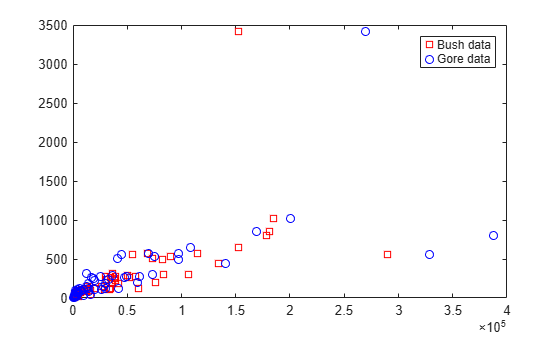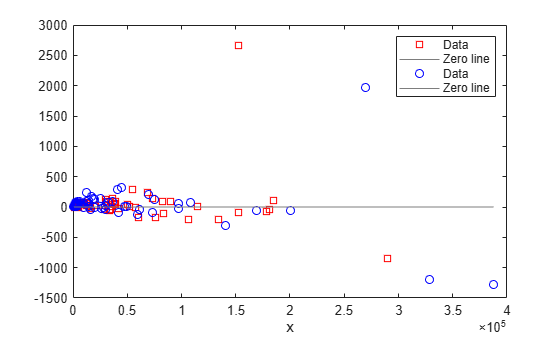# excludedata

Exclude data from fit

## Syntax

``tf = excludedata(x,y,'box',box)``
``tf = excludedata(x,y,'domain',domain)``
``tf = excludedata(x,y,'range',range)``
``tf = excludedata(x,y,'indices',indices)``

## Description

example

````tf = excludedata(x,y,'box',box)` returns a logical array that indicates which elements are outside the box in the xy-plane specified by `box`. The elements of `tf` equal 1 for data points outside the box and 0 for data points inside the box. To exclude data when fitting a curve using `fit`, specify `tf` as the `'Exclude'` value.```

example

````tf = excludedata(x,y,'domain',domain)` identifies data points that have `x`-values outside the interval `domain`.```

example

````tf = excludedata(x,y,'range',range)` identifies the data points with `y`-values outside the interval `range`.```
````tf = excludedata(x,y,'indices',indices)` identifies the data points with indices equal to `indices`.```

## Examples

collapse all

Visualize exclusion rules using random data.

Generate random `x` and `y` data.

```xdata = -3 + 6*rand(1,1e4); ydata = -3 + 6*rand(1,1e4);```

As an example, exclude data that is either inside the box `[-1 1 -1 1]` or outside the domain `[-2 2]`.

```outliers1 = ~excludedata(xdata,ydata,'box',[-1 1 -1 1]); outliers2 = excludedata(xdata,ydata,'domain',[-2 2]); outliers = outliers1|outliers2;```

Plot the data that is not excluded. The white area corresponds to regions that are excluded.

```plot(xdata(~outliers),ydata(~outliers),'.') axis([-3 3 -3 3]) axis square```Load the vote counts and county names for the state of Florida from the 2000 U.S. presidential election.

`load flvote2k`

Use the vote counts for the two major party candidates, Bush and Gore, as predictors for the vote counts for the third-party candidate Buchanan, and plot the scatters:

```plot(bush,buchanan,'rs') hold on plot(gore,buchanan,'bo') legend('Bush data','Gore data')```Assume a model where a fixed proportion of Bush or Gore voters choose to vote for Buchanan.

`f = fittype({'x'})`
```f = Linear model: f(a,x) = a*x ```

Exclude the data from absentee voters, who did not use the controversial “butterfly” ballot.

`nobutterfly = strcmp(counties,'Absentee Ballots');`

Perform a bisquare weights robust fit of the model to the two data sets, excluding absentee voters.

```bushfit = fit(bush,buchanan,f,'Exclude',nobutterfly,'Robust','on'); gorefit = fit(gore,buchanan,f,'Exclude',nobutterfly,'Robust','on');```

Robust fits give outliers a low weight, so large residuals from a robust fit can be used to identify the outliers.

```figure plot(bushfit,bush,buchanan,'rs','residuals') hold on plot(gorefit,gore,buchanan,'bo','residuals')```Calculate the residuals.

```bushres = buchanan - feval(bushfit,bush); goreres = buchanan - feval(gorefit,gore);```

Identify large residuals as those outside the range [-500 500].

```bushoutliers = excludedata(bush,bushres,'range',[-500 500]); goreoutliers = excludedata(gore,goreres,'range',[-500 500]);```

Display the counties corresponding to the outliers. Miami-Dade and Broward counties correspond to the largest predictor values. Palm Beach county, the only county in the state to use the “butterfly” ballot, corresponds to the largest residual values.

`counties(bushoutliers)`
```ans = 2x1 cell {'Miami-Dade'} {'Palm Beach'} ```
`counties(goreoutliers)`
```ans = 3x1 cell {'Broward' } {'Miami-Dade'} {'Palm Beach'} ```

## Input Arguments

collapse all

Data sites of data values, specified as a numeric vector.

Data values, specified as a numeric vector.

Box to find data outside of, specified as a numeric vector ```[xmin xmax ymin ymax]``` with four elements.

Example: [-1 1 0 2]

Domain to find data outside of, specified as a numeric vector ```[xmin xmax]``` with two elements.

Example: [-1 1]

Range to find data outside of, specified as a numeric vector ```[ymin ymax]``` with two elements.

Example: [3 4]

Indices of data points to find, specified as a numeric vector.

Example: [3 7 9]